# torchfilter.base¶

Base classes for filtering.

## Package Contents¶

### Classes¶

 DynamicsModel Base class for a generic differentiable dynamics model, with additive white Filter Base class for a generic differentiable state estimator. KalmanFilterBase Base class for a generic Kalman-style filter. Parameterizes beliefs with a mean KalmanFilterMeasurementModel Helper class that provides a standard way to create an ABC using ParticleFilterMeasurementModel Observation model base class for a generic differentiable particle ParticleFilterMeasurementModelWrapper Helper class for creating a particle filter measurement model (states, VirtualSensorModel Virtual sensor base class for our differentiable Kalman filters.
class torchfilter.base.DynamicsModel(*, state_dim: int)[source]

Bases: torch.nn.Module, abc.ABC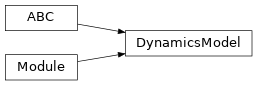Base class for a generic differentiable dynamics model, with additive white Gaussian noise.

Subclasses should override either forward or forward_loop for computing dynamics estimates.

state_dim

Dimensionality of our state.

Type

int

forward(self, *, initial_states: types.StatesTorch, controls: types.ControlsTorch) → Tuple[types.StatesTorch, types.ScaleTrilTorch][source]

Dynamics model forward pass, single timestep.

Computes both predicted states and uncertainties. Note that uncertainties correspond to the (Cholesky decompositions of the) “Q” matrices in a standard linear dynamical system w/ additive white Gaussian noise. In other words, they should be lower triangular and not accumulate – the uncertainty at at time t should be computed as if the estimate at time t - 1 is a ground-truth input.

By default, this is implemented by bootstrapping the forward_loop() method.

Parameters
• initial_states (torch.Tensor) – Initial states of our system. Shape should be (N, state_dim).

• controls (dict or torch.Tensor) – Control inputs. Should be either a dict of tensors or tensor of size (N, ...).

Returns

Tuple[torch.Tensor, torch.Tensor] – Predicted states & uncertainties. States should have shape (N, state_dim), and uncertainties should be lower triangular with shape (N, state_dim, state_dim).

forward_loop(self, *, initial_states: types.StatesTorch, controls: types.ControlsTorch) → Tuple[types.StatesTorch, torch.Tensor][source]

Dynamics model forward pass, over sequence length T and batch size N. By default, this is implemented by iteratively calling forward().

Computes both predicted states and uncertainties. Note that uncertainties correspond to the (Cholesky decompositions of the) “Q” matrices in a standard linear dynamical system w/ additive white Gaussian noise. In other words, they should be lower triangular and not accumulate – the uncertainty at at time t should be computed as if the estimate at time t - 1 is a ground-truth input.

To inject code between timesteps (for example, to inspect hidden state), use register_forward_hook().

Parameters
• initial_states (torch.Tensor) – Initial states to pass to our dynamics model. Shape should be (N, state_dim).

• controls (dict or torch.Tensor) – Control inputs. Should be either a dict of tensors or tensor of size (T, N, ...).

Returns

Tuple[torch.Tensor, torch.Tensor] – Predicted states & uncertainties. States should have shape (T, N, state_dim), and uncertainties should be lower triangular with shape (T, N, state_dim, state_dim).

jacobian(self, initial_states: types.StatesTorch, controls: types.ControlsTorch) → torch.Tensor[source]

Returns Jacobian of the dynamics model.

Parameters
• states (torch.Tensor) – Current state, size (N, state_dim).

• controls (dict or torch.Tensor) – Control inputs. Should be either a dict of tensors or tensor of size (N, ...).

Returns

torch.Tensor – Jacobian, size (N, state_dim, state_dim)

class torchfilter.base.Filter(*, state_dim: int)[source]

Bases: torch.nn.Module, abc.ABC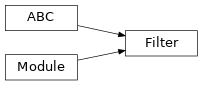Base class for a generic differentiable state estimator.

As a minimum, subclasses should override:

• initialize_beliefs for populating the initial belief of our estimator

• forward or forward_loop for computing state predictions

state_dim

Dimensionality of our state.

Type

int

abstract initialize_beliefs(self, *, mean: types.StatesTorch, covariance: types.CovarianceTorch) → None[source]

Initialize our filter with a Gaussian prior.

Parameters
• mean (torch.Tensor) – Mean of belief. Shape should be (N, state_dim).

• covariance (torch.Tensor) – Covariance of belief. Shape should be (N, state_dim, state_dim).

forward(self, *, observations: types.ObservationsTorch, controls: types.ControlsTorch) → types.StatesTorch[source]

Filtering forward pass, over a single timestep.

By default, this is implemented by bootstrapping the forward_loop() method.

Parameters
• observations (dict or torch.Tensor) – Observation inputs. Should be either a dict of tensors or tensor of size (N, ...).

• controls (dict or torch.Tensor) – Control inputs. Should be either a dict of tensors or tensor of size (N, ...).

Returns

torch.Tensor – Predicted state for each batch element. Shape should be (N, state_dim).

forward_loop(self, *, observations: types.ObservationsTorch, controls: types.ControlsTorch) → types.StatesTorch[source]

Filtering forward pass, over sequence length T and batch size N. By default, this is implemented by iteratively calling forward().

To inject code between timesteps (for example, to inspect hidden state), use register_forward_hook().

Parameters
• observations (dict or torch.Tensor) – observation inputs. Should be either a dict of tensors or tensor of size (T, N, ...).

• controls (dict or torch.Tensor) – control inputs. Should be either a dict of tensors or tensor of size (T, N, ...).

Returns

torch.Tensor – Predicted states at each timestep. Shape should be (T, N, state_dim).

class torchfilter.base.KalmanFilterBase(*, dynamics_model: DynamicsModel, measurement_model: KalmanFilterMeasurementModel, **unused_kwargs)[source]

Bases: torchfilter.base.Filter, abc.ABC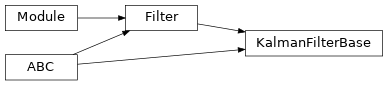Base class for a generic Kalman-style filter. Parameterizes beliefs with a mean and covariance.

Subclasses should override _predict_step() and _update_step().

dynamics_model

Forward model.

Type

torchfilter.base.DynamicsModel

measurement_model

Measurement model.

Type

torchfilter.base.KalmanFilterMeasurementModel

forward(self, *, observations: types.ObservationsTorch, controls: types.ControlsTorch) → types.StatesTorch[source]

Kalman filter forward pass, single timestep.

Parameters
• observations (dict or torch.Tensor) – Observation inputs. Should be either a dict of tensors or tensor of shape (N, ...).

• controls (dict or torch.Tensor) – Control inputs. Should be either a dict of tensors or tensor of shape (N, ...).

Returns

torch.Tensor – Predicted state for each batch element. Shape should be (N, state_dim).

initialize_beliefs(self, *, mean: types.StatesTorch, covariance: types.CovarianceTorch) → None[source]

Set filter belief to a given mean and covariance.

Parameters
• mean (torch.Tensor) – Mean of belief. Shape should be (N, state_dim).

• covariance (torch.Tensor) – Covariance of belief. Shape should be (N, state_dim, state_dim).

property belief_mean(self) → types.StatesTorch

Posterior mean. Shape should be (N, state_dim).

property belief_covariance(self) → types.CovarianceTorch

Posterior covariance. Shape should be (N, state_dim, state_dim).

class torchfilter.base.KalmanFilterMeasurementModel(*, state_dim, observation_dim)[source]

Bases: abc.ABC, torch.nn.Module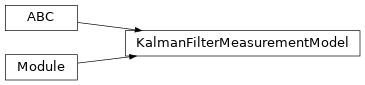Helper class that provides a standard way to create an ABC using inheritance.

state_dim

State dimensionality.

Type

int

observation_dim

Observation dimensionality.

Type

int

abstract forward(self, *, states: types.StatesTorch) → Tuple[types.ObservationsNoDictTorch, types.ScaleTrilTorch][source]

Observation model forward pass, over batch size N.

Parameters

states (torch.Tensor) – States to pass to our observation model. Shape should be (N, state_dim).

Returns

Tuple[torch.Tensor, torch.Tensor] – tuple containing expected observations and cholesky decomposition of covariance. Shape should be (N, M).

jacobian(self, *, states: types.StatesTorch) → torch.Tensor[source]

Returns Jacobian of the measurement model.

Parameters

states (torch.Tensor) – Current state, size (N, state_dim).

Returns

torch.Tensor – Jacobian, size (N, observation_dim, state_dim)

class torchfilter.base.ParticleFilterMeasurementModel(state_dim: int)[source]

Bases: abc.ABC, torch.nn.Module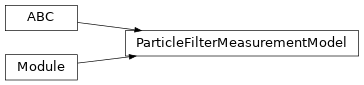Observation model base class for a generic differentiable particle filter; maps (state, observation) pairs to the log-likelihood of the observation given the state ( $$\log p(z | x)$$ ).

state_dim

Dimensionality of our state.

Type

int

abstract forward(self, *, states: types.StatesTorch, observations: types.ObservationsTorch) → torch.Tensor[source]

Observation model forward pass, over batch size N. For each member of a batch, we expect M separate states (particles) and one unique observation.

Parameters
• states (torch.Tensor) – States to pass to our observation model. Shape should be (N, M, state_dim).

• observations (dict or torch.Tensor) – Measurement inputs. Should be either a dict of tensors or tensor of size (N, ...).

Returns

torch.Tensor – Log-likelihoods of each state, conditioned on a corresponding observation. Shape should be (N, M).

class torchfilter.base.ParticleFilterMeasurementModelWrapper(kalman_filter_measurement_model: KalmanFilterMeasurementModel)[source]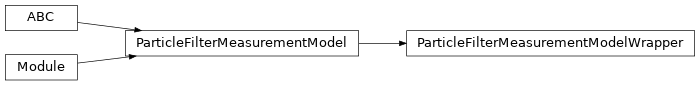Helper class for creating a particle filter measurement model (states, observations -> log-likelihoods) from a Kalman filter one (states -> observations).

Parameters

kalman_filter_measurement_model (torchfilter.base.KalmanFilterMeasurementModel) – Kalman filter measurement model instance to wrap.

forward(self, *, states: types.StatesTorch, observations: types.ObservationsTorch) → torch.Tensor[source]

Observation model forward pass, over batch size N. For each member of a batch, we expect M separate states (particles) and one unique observation.

Parameters
• states (torch.Tensor) – States to pass to our observation model. Shape should be (N, M, state_dim).

• observations (torch.Tensor) – Measurement inputs. Should be either a dict of tensors or tensor of size (N, ...).

Returns

torch.Tensor – Log-likelihoods of each state, conditioned on a corresponding observation. Shape should be (N, M).

class torchfilter.base.VirtualSensorModel(state_dim: int)[source]

Bases: abc.ABC, torch.nn.Module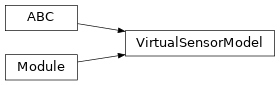Virtual sensor base class for our differentiable Kalman filters.

Maps each observation input to a predicted state and uncertainty, in the style of BackpropKF. This is often necessary for complex observation spaces like images or point clouds, where it’s not possible to learn a standard state->observation measurement model.

state_dim

Dimensionality of our state.

Type

int

abstract forward(self, *, observations: types.ObservationsTorch) → Tuple[types.StatesTorch, types.ScaleTrilTorch][source]

Predicts states and uncertainties from observation inputs.

Uncertainties should be lower-triangular Cholesky decompositions of covariance matrices.

Parameters

observations (dict or torch.Tensor) – Measurement inputs. Should be either a dict of tensors or tensor of size (N, ...).

Returns

Tuple[torch.Tensor, torch.Tensor] – Predicted states & uncertainties. States should have shape (N, state_dim), and uncertainties should be lower triangular with shape (N, state_dim, state_dim).